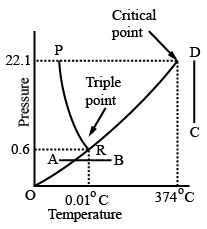# ISRO Scientist or Engineer Mechanical 2009

Instructions

For the following questions answer them individually

Question 11

# The transition Reynolds number for flow over a flat plate is $$5 * 10^5.$$ What is the distance from the leading edge at which transition will occur for flow of water with a uniform velocity of 1m/s? (For water, the kinematic viscosity, $$v = 0.86 * 10^{-6} m^2/s)$$Question 12

# For a reciprocating water pump having cylinder dia d and crank radius r, if W is the weight of water lifted, the coefficient of discharge isQuestion 13

# Both free vortex and forced vortex can be expressed mathematically in terms of tangential velocity V at the corresponding radius r. Choose the correct combinationQuestion 14

# A bucket of water is hanging from a spring balance. An iron piece is suspended into water without touching sides of bucket from another support. The spring balance reading willQuestion 15

# A single-stage impulse turbine with a diameter of 120 cm runs at 3000 rpm. If the blade speed ratio is 0.42, then, the inlet velocity of steam will beQuestion 16

# The critical point and triple point data for water are, $$T_c = 374^\circ C, P_c = 22.1 Mpa, T_T = 0.01^\circ C, P_T = 0.6 kPa$$ Indicate the phase change that will occur in following cases,(i)Ice at 0.5 kPa is heated isobarically(ii) Water vapour at $$400^\circ C$$ is compressed isothermallyQuestion 17

# The ratio of specific heats of a gas is 1.4 and the value of specific heat at constant pressure is $$7.0 cal/mole^\circ C$$. The difference between specific heat at constant pressure and specific heat at constant volume will be $$(in cal /mole^\circ C)$$Question 18

# The coefficient of linear expansion of a solid is L. The volume V of a cube of this solid on being heated by $$1^\circ C$$ will change byQuestion 19

# Two springs of equal length but having stiffness of 10 N/mm and 15 N/mm support a mass of 2 tonnes in series. Find the frequency of vibrationQuestion 20

# Ratio of pitch circle diameter in millimeters to the number of teeth, is known asOR